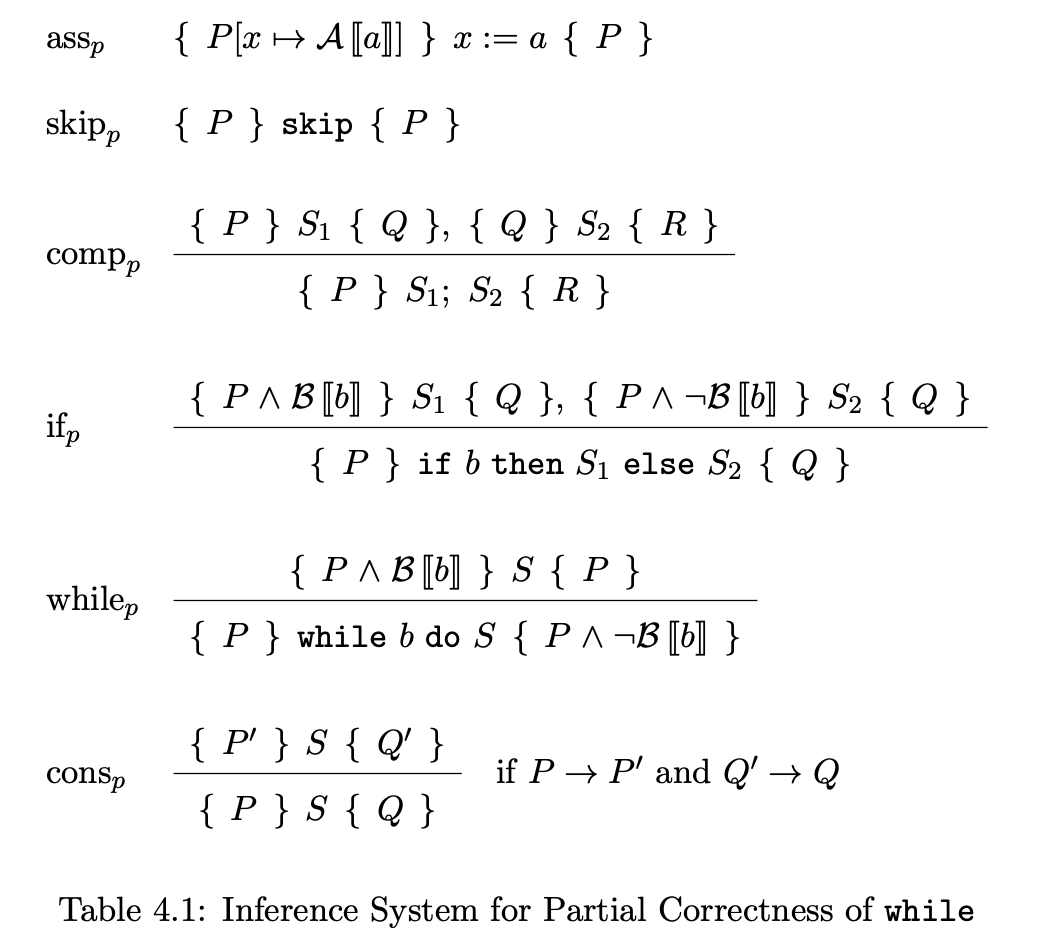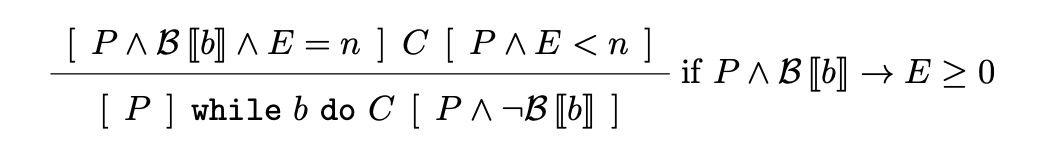# Ch6 计算理论 计算正确性

## Proving correctness

Posted by R1NG on May 5, 2021 Viewed Times

# 6. 计算正确性

## 完全正确性和部分正确性

$\text{Actual}(P) \equiv \{<\sigma_{start}, \sigma_{end}> ~\vert~ <P, \sigma_{start}> \Rightarrow \sigma_{end}\}$

$\text{Specification}(\text{Pre}, \text{Post}) \equiv \{<\sigma_{start}, \sigma_{end}> ~\vert~ <\text{Pre}(\sigma_{start}) \Rightarrow \text{Post}(\sigma_{end})\}.$

$\text{Specification}(\text{Pre}, \text{Post}) \subseteq \text{Actual}(P).$

## 霍尔逻辑: 描述部分正确性## 霍尔逻辑: 描述完全正确性## 正确性证明的技巧及方法

$S_1; ~ (\text{while} ~ b ~\text{do}~ S_2); ~ S_3$

1. 首先, 寻找或构造出循环体的循环不变量 $R$. 并使用基本规则推导
$\{P\} ~ S_1 ~ \{R\}$

$\{R \wedge \neg b\} ~ S_1 ~ \{Q\}.$

1. 在得到 前部程序体 和 后部程序体 这两个部分的正确性证明后, 我们对循环体
$\{R \wedge b\} ~S_2~ \{R\}$

1. 最后, 对表示循环体的霍尔三元组应用 $\text{while}$ 规则, 再使用 $\text{comp}$ 规则将三者联结起来, 证毕.

1. 循环不变量必须包含所有循环体在循环中更新 并在循环主体之后或在后置条件中引用的变量.
2. 我们所希望在循环结束后仍然为真的条件必须包含在循环不变量中.
3. 循环不变量中需要包含程序中所使用的常量.
4. 后置条件的形式往往和循环不变量保持一致. 比如: 若后置条件是一个不等式, 我们可以尝试将后置条件这个不等式直接作为循环不变量.
5. 循环不变量的真伪不会被循环体的执行与否而改变.